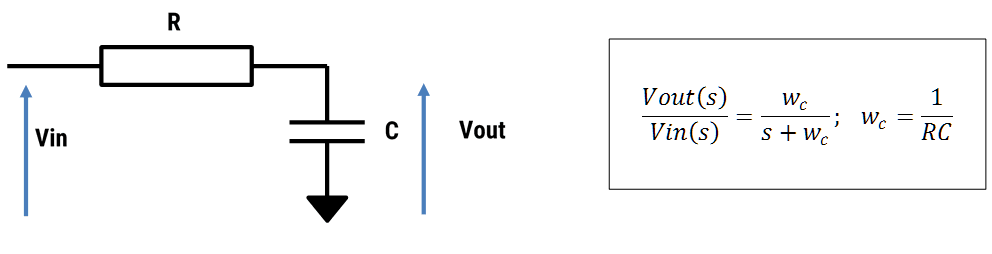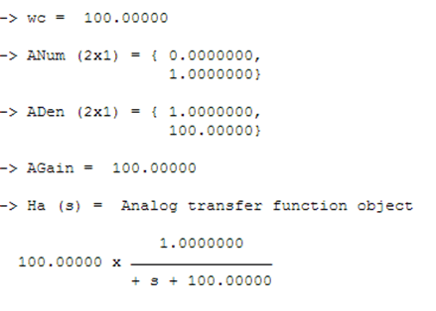# Analog transfer function

Syntax
Ha=analogtf(ANum, ADen, AGain, DFormat)

Description
Define an analog filter object. Where, the ANum and ADen vectors are the Laplace transfer function coefficients in descending order.

Examples
A first order analog lowpass filter may be designed by an RC network:Choosing R=100k$$Omega$$ and C=100nF, gives cut-off at 100rad/s or ≈ 15.9Hz. The analog transfer function may be simply implemented in FilterScript (output shown below) as:

 Main()

wc=100;  // 1/(R*C);
ANum={0,1};